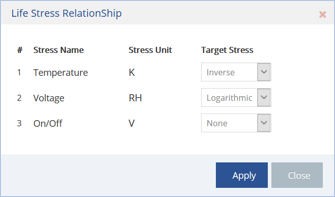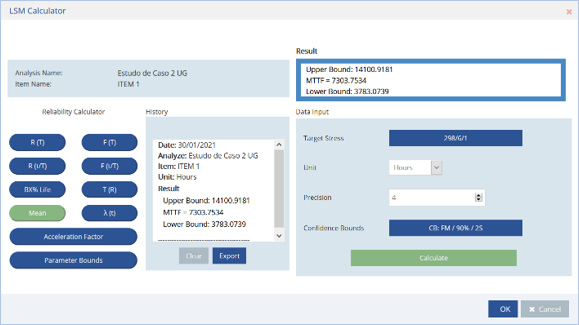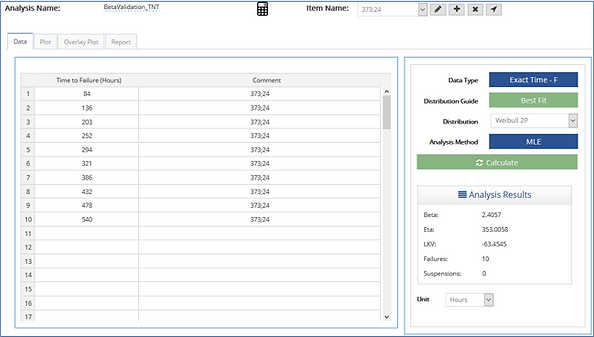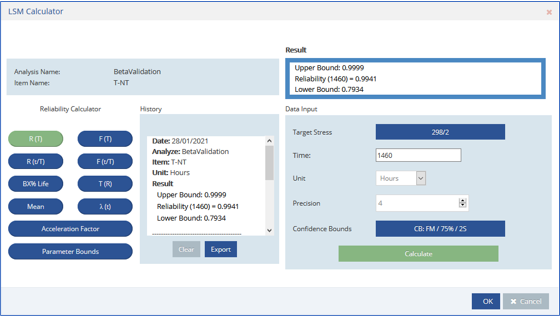top of page

# USER GUIDE

## 7.1 Case Study 1: Arrhenius

A company is installing a new server in one geographic region; however, the product will be scattered across other different geographic regions. As each region possesses a different temperature range, this server is being designed to have a minimum reliability of 85% for a warranty period of 2 years to its worst temperature condition (with an 80% unilateral confidence bound).

The highest registered temperature in the hottest region was 42.5 degrees Celsius. To ensure the new server will meet the reliability requirements, field-controlled tests were conducted in a motherboard sample, in different temperatures.

The table below contains the time to failures and the respective temperatures at which each item was tested.As the given temperature data is in Kelvin, the desired target stress of 42.5 degrees Celsius must be converted to 315.5 degrees Kelvin.The best fit for this sample data was the Weibull distribution, and since we are treating temperature only, the Arrhenius model was chosen. The Target Stress Probability Plot for a stress target of 315.5 K was made with a top one-sided confidence bound of 80% confidence to analyze the worst scenario for this bound over a period of 17,520 hours (2-year operation).It seems that the probability of failure on the top unilateral bound is close to 14%, and so these motherboards meet their reliability requirements. However, as a special Weibull scale plot is a little difficult to read precisely, let us calculate the reliability for a 2-year non-stop operation period.With a reliability of 85.27%, the motherboard has met its reliability requirements.

## 7.2 Case Study 2: Multiple Stress Types

An electronic device of an automatic system was submitted to a test while the following conditions were controlled: temperature, voltage, and operation (on-off/continuous). The main goal of this test is to determine the reliability of this device for a 3-year operating period. In normal conditions, this device will be operating 8 hours a day for every day of the week, including weekends, considering regular atmospheric conditions and a voltage of 6V as the normal operating conditions.

The next table contains the data referring to the times of failure of the tested samples and the conditions in which each of them operated during the test.There are more than two stress types, so, in this case, the General Log-Linear stress model is mandatory. It is important to note that one of them is a discrete variable and even if there were no other stress type, only the General Log-Linear can fit discrete variables on its formulation.

On the “Data Type” button, the sheet was configured to receive grouped and suspended data, as well as the stress types.As the discrete random variable is only represented in binary values (on/off) it has no unit, so the user can enter an arbitrary name for this field. Here, it was chosen a DRV (Discrete Random Variable) to represent the unit of this variable.

The standard atmospheric conditions consider a temperature of 298 Kelvin, the target voltage is 6V, and as for the on-off/continuous condition, the target stress is achieved when the device is on (DRV = 1).The best fit was the Weibull distribution, and, for the stress transformation, it was set an inverse transformation for the temperature, a logarithmic transformation for the voltage and no transformation was used to treat the discrete variable on/off (when this option is set, a standard exponential function will model the stress).For a 3-year period, the total operation time, in hours, will be:

3 * 365 * 8 = 8760 hours

The Mean Life and B10 life were calculated for the usage condition (Temperature = 298 Kelvin, Voltage = 6 Volts and Operating full time) in the LSM calculator:

Mean LifeB10 LifeIn the next plot, it is possible to see the life distribution for each individual stress set combination and for the Target Stress (usage condition).Now with the model created, the company can estimate the reliability for any target stress combination (different usage condition in different regions) and verify how the product will perform.

## 7.3 Case Study 3: Validating Beta Parameter

A CPU was submitted to a controlled test, and two stress factors were applied: the environment temperature and the battery voltage. This CPU will be used in a server cluster and will constantly be refrigerated to operate in an environment of 25 degrees Celsius and its source voltage will be 2V. It is estimated that this CPU will operate only when requested (2 hours per day).

The engineers want first to validate the test before calculating the reliability for a 2-year period.To validate a test, a comparison of the beta parameter of each sample group was conducted. The data were divided in three groups, and an LDA was applied to each one. It is important to note that to validate the test, all sample groups must be analyzed using the same distribution and the same parameter estimation method.

Note: as the objective in the first part of the case study is just compare the beta parameter to validate the stress test sample, the LDA module was applied to perform the life distribution for each stress combination.

The next images were generated on the LDA module.As shown in the results of each LDA performed, changing the temperature value reflected significantly more on the beta parameter than doubling the voltage. There is little variation in this parameter; but, in the contour plot, it is possible to visualize how similar the betas are.For a 90% confidence bound, the contour plots show small variance in the beta values of each sample group. Therefore, this test can be validated.

Now, returning to the LSM module, the data can be treated using a T-NT stress model (recommended to treat thermal/non-thermal stress).Setting the target stress to 298 K (standard atmospheric temperature) and 2V given by the battery in the normal condition, and knowing that this item operates 2 hours per day (1460 hours for a 2-year period), the reliability required can be estimated using the calculator:Chapter 7: LSM Case Studies
7.1 Case Study 1: Arrhenius
7.2 Case Study 2: Multiple Stress Types
7.3 Case Study 3: Validating Beta Parameter
bottom of page